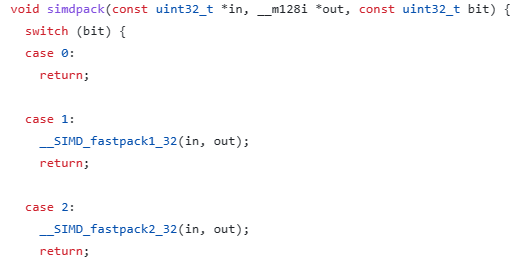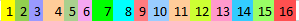## Integer compressionUnderstanding Simd Compression by Lemire

time to read 4 min | 728 words

In the previous post, I showed how you can use integer compression using variable-size integers. That is a really straightforward approach for integer compression, but it isn’t ideal. To start with, it means that we take at least one byte for each number, but in many cases, especially if we are using delta encoding, we can pack things a lot more tightly. For reference, here are the first 15 records we have in the posting list I’m using for testing, delta encoded:

1871143144
4
4
4
4
4
4
4
4
4
4
7984
4
4
4

See the range of 4, repeating. Each one of them can be represented by 3 bits, but we’ll waste 32 – 64 bits to hold it.

When you are searching for data on “database stuff”, the building blocks that are being used to build databases, you’ll usually find Lemire there. In this case, I would like to talk about the SimdComp library. From the GitHub repository:

A simple C library for compressing lists of integers using binary packing and SIMD instructions. This library can decode at least 4 billion of compressed integers per second on most desktop or laptop processors.

Those are some really nice numbers, but I actually have a pet peeve with the name of the library. It isn’t actually doing compression, what it does is bit packing, which is something quite different.

Bit packing just takes numbers that are 32 bits in length and stores them using fewer bits.  For example, let’s consider the following list: 1,2,3,4,5,6,7,8,9,10. If I would store that in 32 bits integers, that would take 40 bytes. But given that the maximum size is 10, I can use 4 bits integers, instead. Taking only 5 bytes.

The core of the library is this set of routines:

• simdpack()
• simdunpack()

All of them have roughly the same structure, something like this:This switch statement goes on for 32 bits. Each time selecting a different function. This code makes a lot of assumptions. You’ll always give it exactly an input of 128 numbers and it will pack them and write them to output. The idea is to be as compact as you possibly can.

Reading this code is.. a challenge, because there is so much mental weight behind it, or so it seems. For example, take a look at how we pack a set of numbers into 2-bit integers:

The actual code goes on for quite a while (104 of dense SIMD code), so let’s try to break it up a bit. If we’ll translate this from SIMD to scalar, we’ll have code like this:

If you are used to manipulating bits, it’s fairly straightforward. We start by setting the output to the first value, then the second value was shifted by 2 bits and added to the value, then the third value was shifted by 4 bits, etc.

With SIMD, the interesting part is that we aren’t operating on a single value, but 4 at a time. That also means that we have a difference in how the data actually end up being packed.

Given a list of values from 1 .. 16, bit packing them will usually result in the data looking like this:In contrast, the SIMD bit packing model will store the data like so:This is a result of operating on 4 items at a time, but in practice, the actual placement of the bits does not matter much, just that we are able to store and retrieve them.

Note that for packing, we have two options, one that uses a mask and the other that does not. That will be important later. The only difference between those options is whether a mask is applied before we splice the bits into the output, nothing else.

The reason I said that this isn’t compression is that this library is focused solely on packing the bits, it has no behavior / concept beyond that stage. In the next post, we’ll start looking into how we can make use of this in more interesting ways.